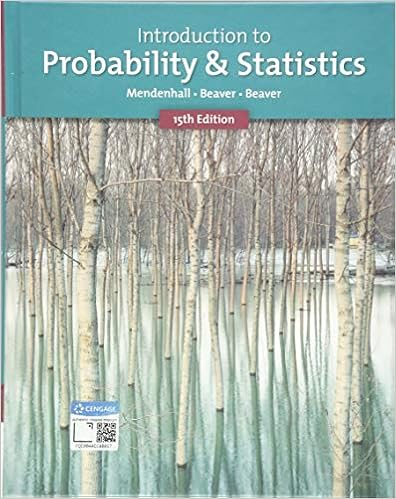# An analyst in michigan surveys a sample of registered

• Homework Help
• 3
• 100% (1) 1 out of 1 people found this document helpful

This preview shows page 2 - 3 out of 3 pages.

##### We have textbook solutions for you!
The document you are viewing contains questions related to this textbook.The document you are viewing contains questions related to this textbook.
Chapter 2 / Exercise 17
Introduction to Probability and Statistics
Beaver/MendenhallExpert Verified
7.An analyst in Michigan surveys a sample of registered Democrats and compares the results with those obtained from a sample of registered Republicans. This would be an example of: A.dependent samples. B.independent samples.
8.In order to compare two scales, 30 objects are weighed on both scales. Each object would then have two weight values (one from scale 1 and one from scale 2). Based on the nature of the differences in the two weight measurements for the 30 objects, the two scales may be compared. Do these samples represent dependent or independent samples?
9.Salt-free diets are often prescribed to people with high blood pressure. The data shown below were obtained from an experiment designed to estimate the reduction in diastolic blood pressure as a result of following a salt-free diet for two weeks. Before: 112 108 97 90 104 89 94 95 After: 107 104 98 90 103 91 94 94 Let d= diastolic blood pressure before diet diastolic blood pressure after diet. Assume diastolic readings to be normally distributed. The sample mean difference of the sample is 0.8 and the sample standard deviation of the difference is 2.348. Test whether a salt-free diet for two weeks is effective in reducing the diastolic blood pressure. Test statistic = _________ 10.100 98 102 96
##### We have textbook solutions for you!
The document you are viewing contains questions related to this textbook.The document you are viewing contains questions related to this textbook.
Chapter 2 / Exercise 17
Introduction to Probability and Statistics
Beaver/MendenhallExpert Verified
•••